Physics Numericals Class 11 Chapter 4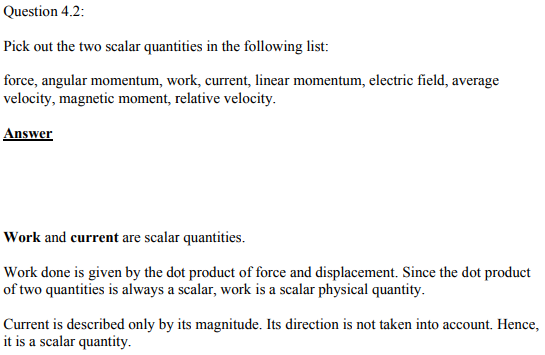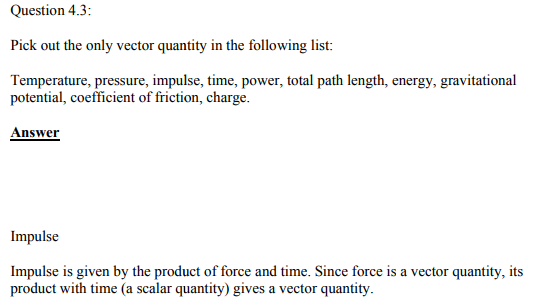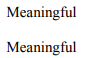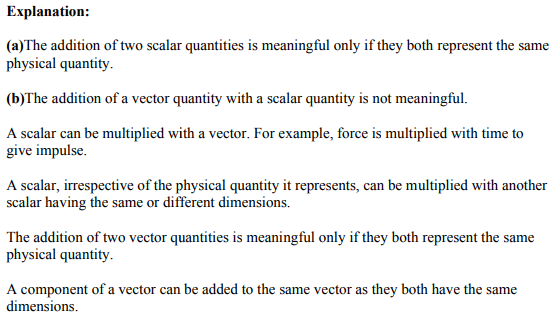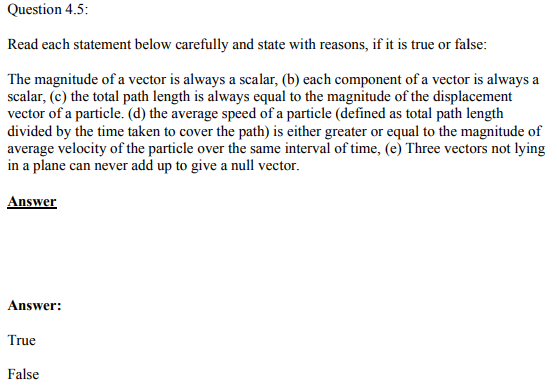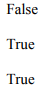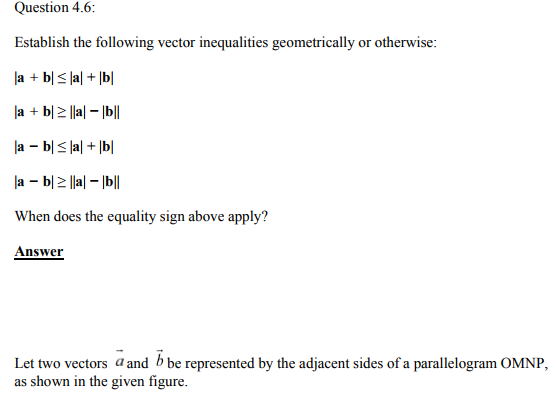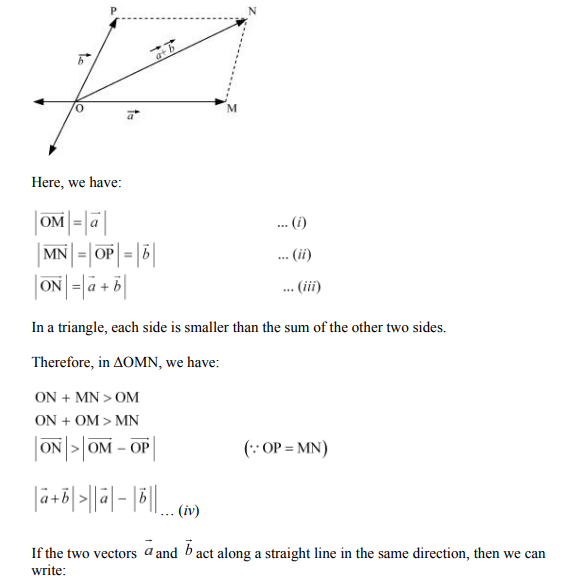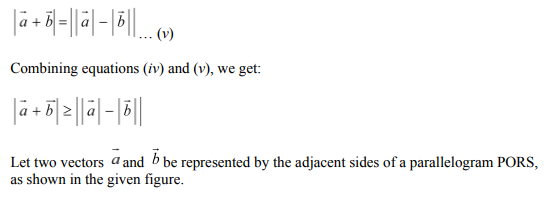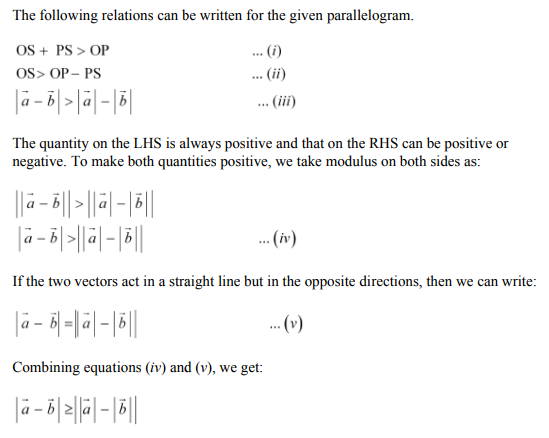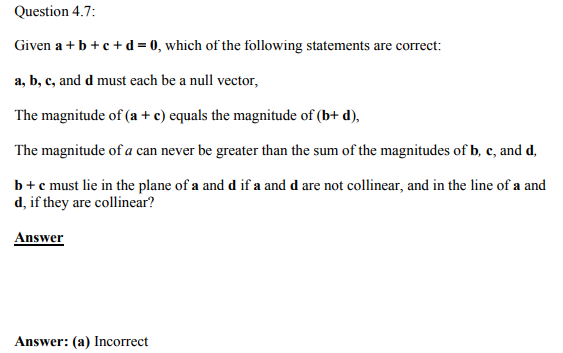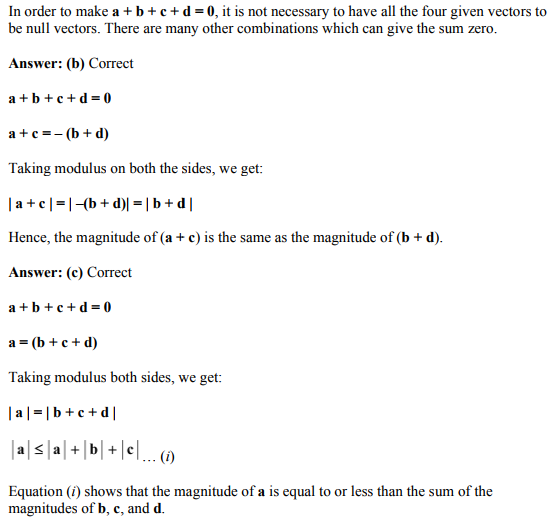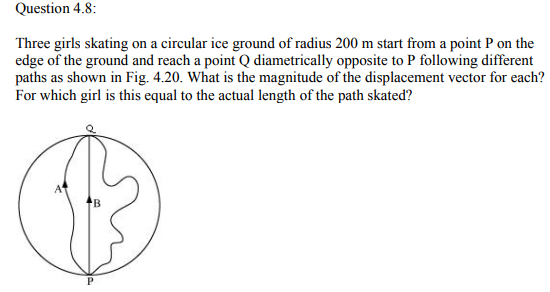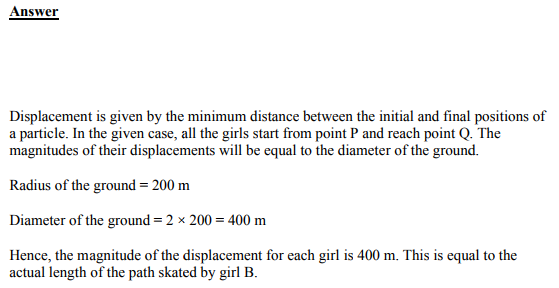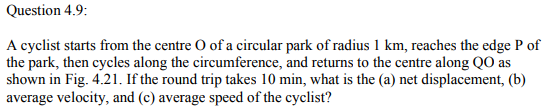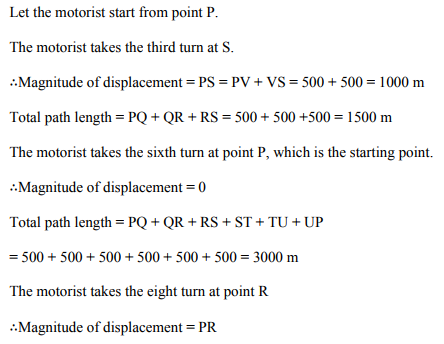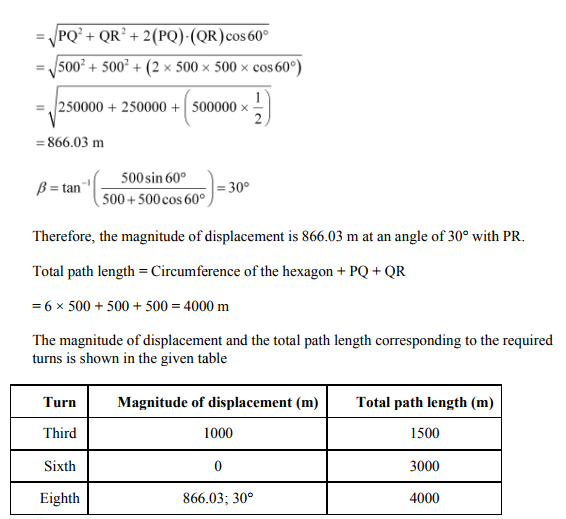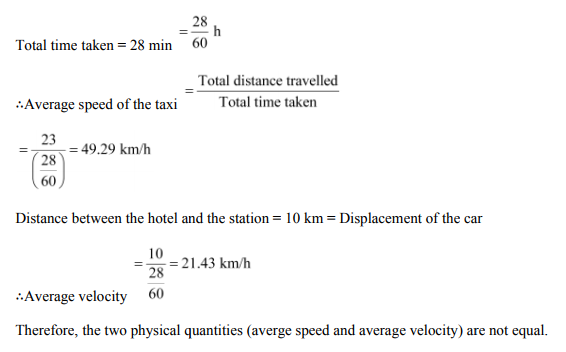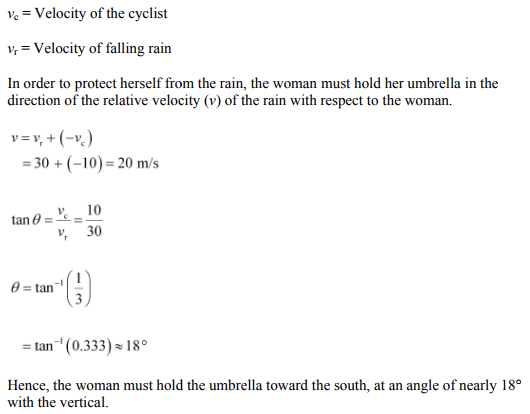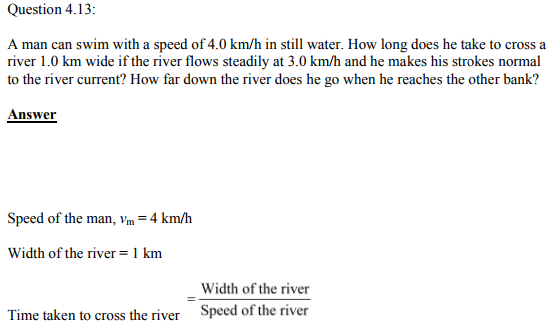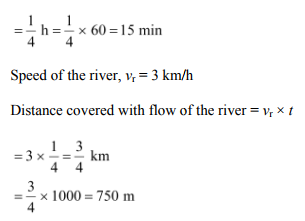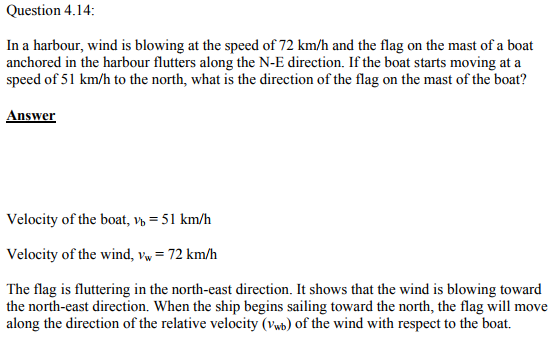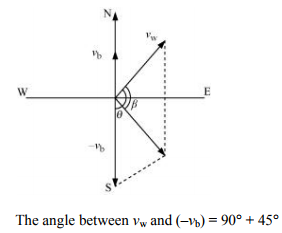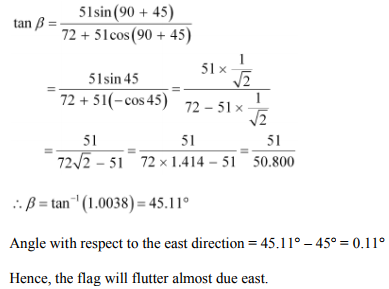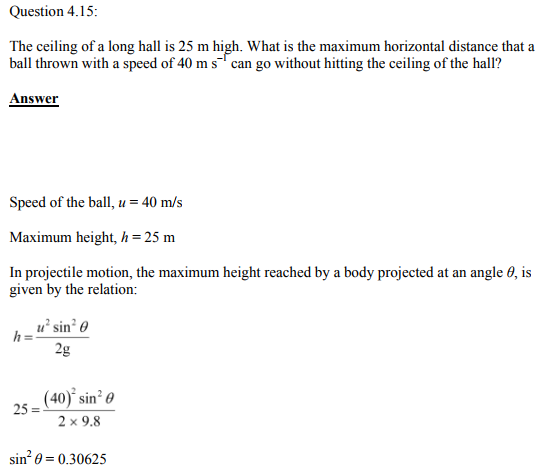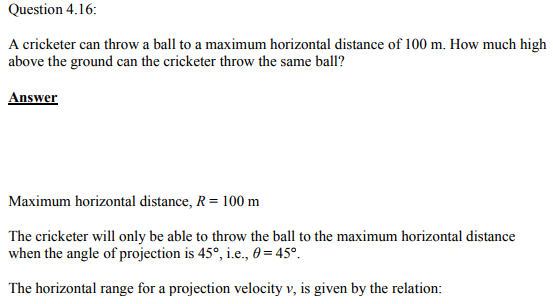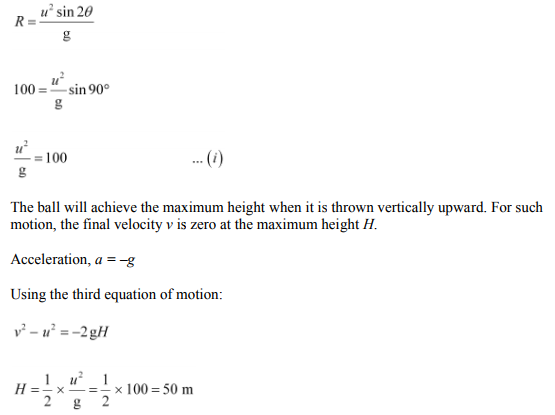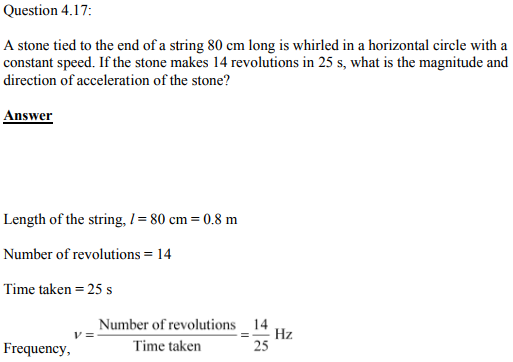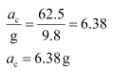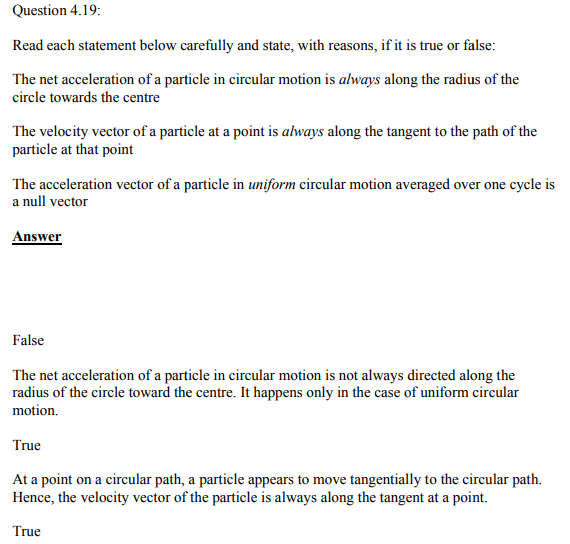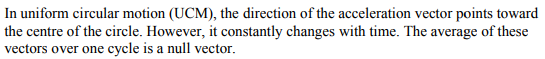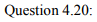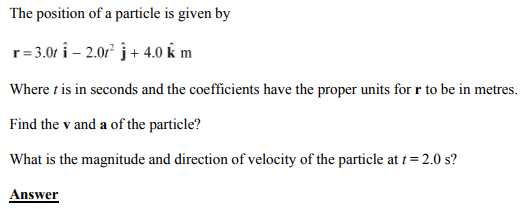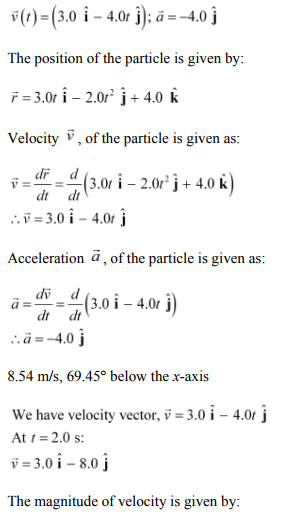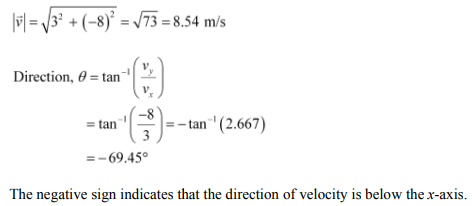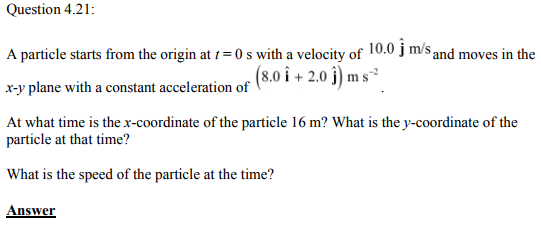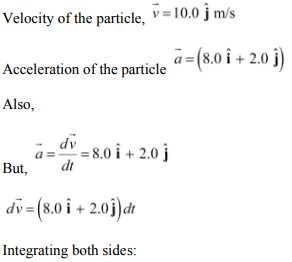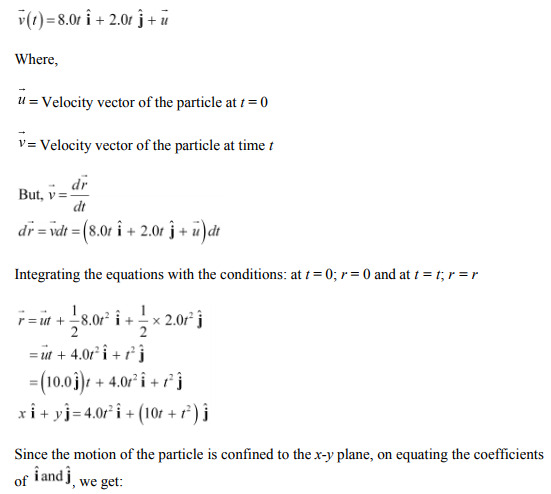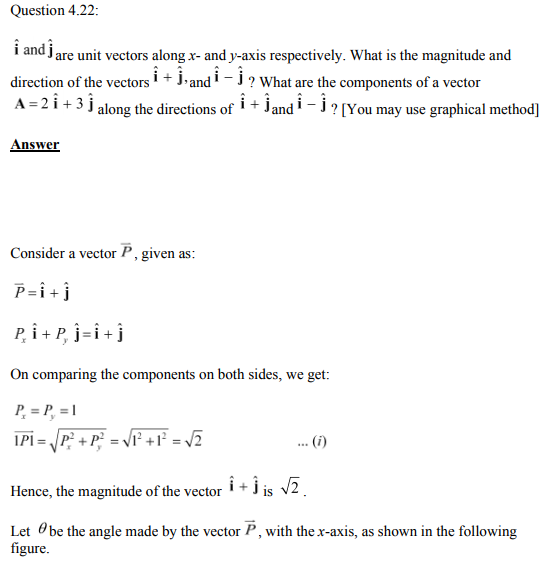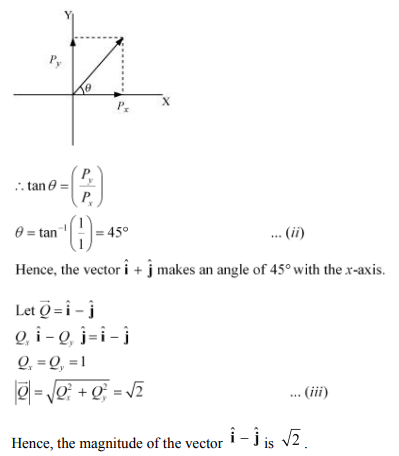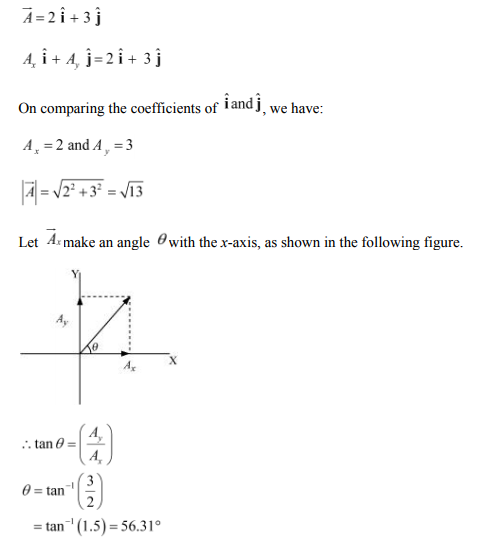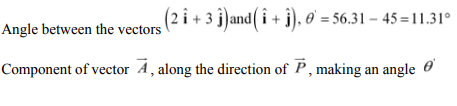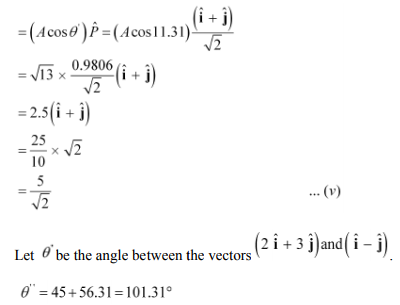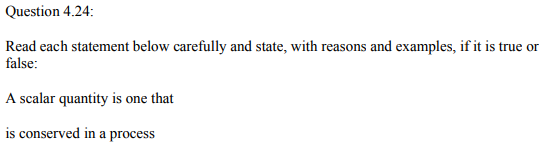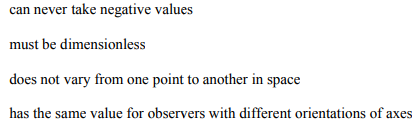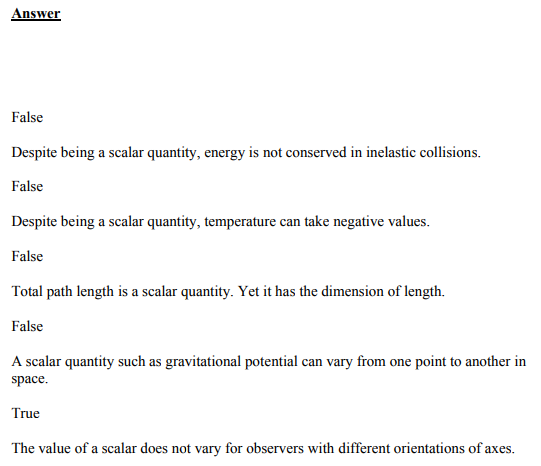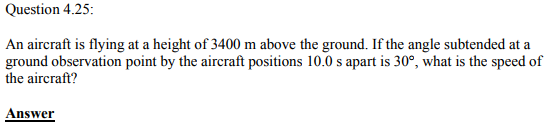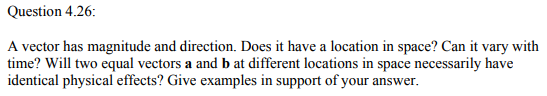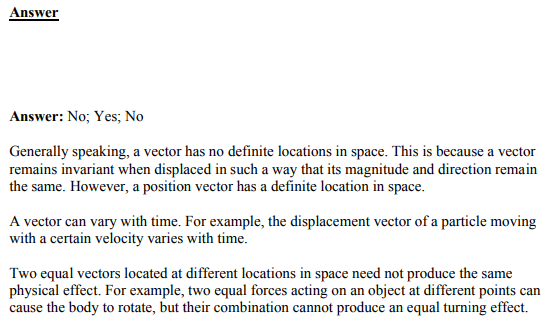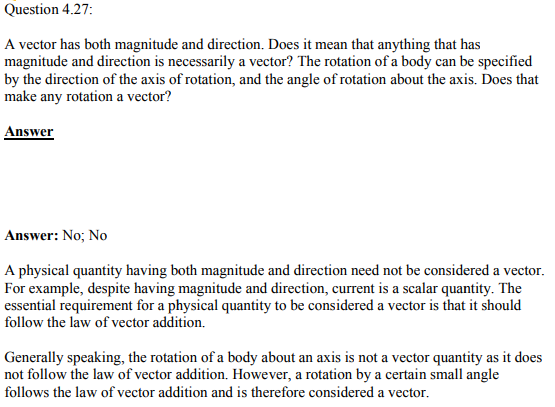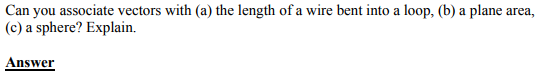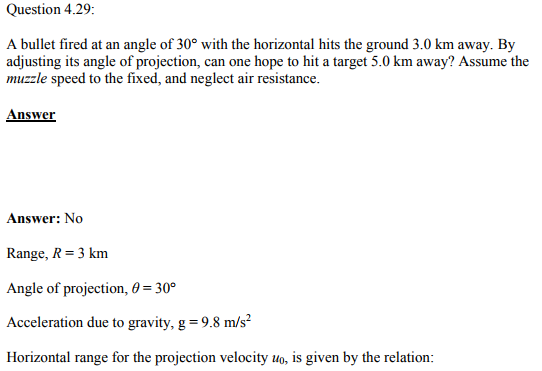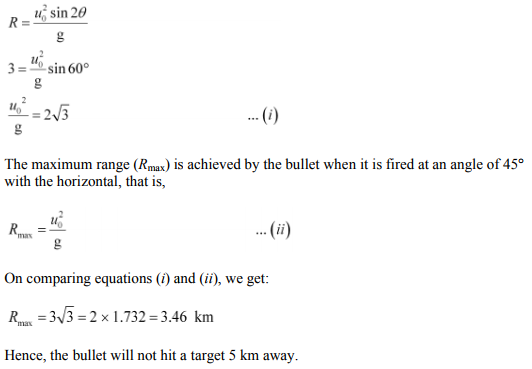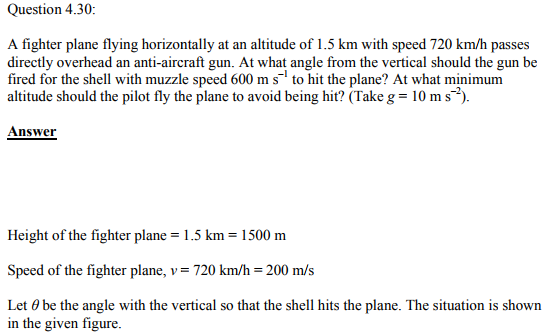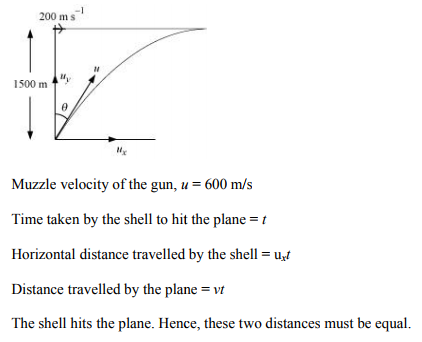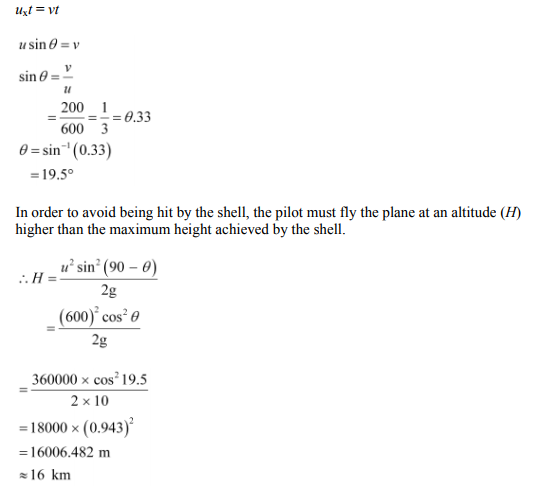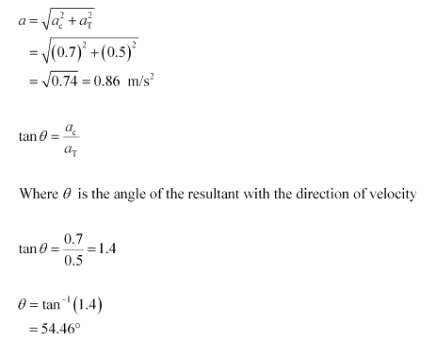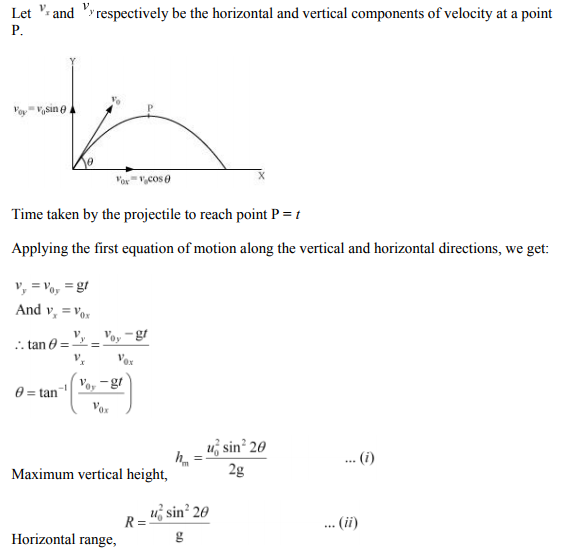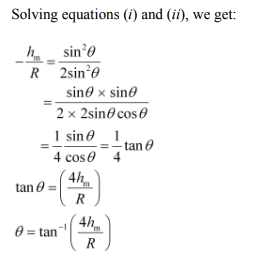Practise This Question

Knowing that mass of Moon is M81 where M is the mass of Earth, find the distance of the point where gravitational field due to Earth and Moon cancel each other, from the Moon. Given that distance between Earth and Moon is 60 R. Where R is the radius of Earth